# Electronics and Communication Engineering - Automatic Control Systems

Exercise : Automatic Control Systems - Section 1
1.
For the system in the given figure the characteristic equation is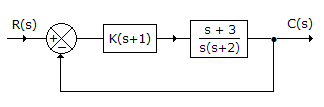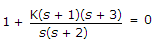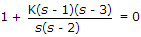K(s + 1)(s + 3) = 0
s(s + 2) = 0
Explanation:

Transfer function =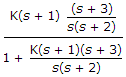.

Hence characteristic equation is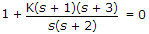.

2.
Mechanical impedance is the ratio of
rms force to rms velocity
rms force to rms displacement
rms velocity to rms displacement
none of the above
Explanation:

In force voltage analogy force is analogous to voltage and velocity to current. Just as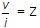, force/velocity = mechanical impedance.

3.
For the system in the given figure. The transfer function C(s)/R(s) is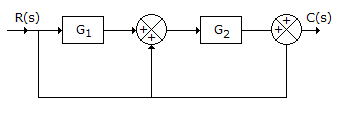G1 + G2 + 1
G1 G2 + 1
G1 G2 + G2 + 1
G1 G2 + G1 + 1
Explanation:

Transfer function = (G1 + 1) G2 + 1 = G1 G2 + G2 + 1.

4.
Whether a linear system is stable or unstable that it
is a property of the system only
depends on the input function only
both (a) and (b)
either (a) or (b)
Explanation:

Stability is a property of the system only.

5.
For the system of the given figure the transfer function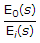=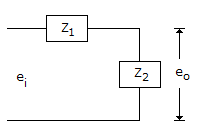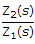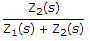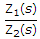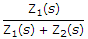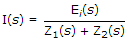, E0(s) = [I(s)][Z2(s)] or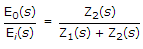.